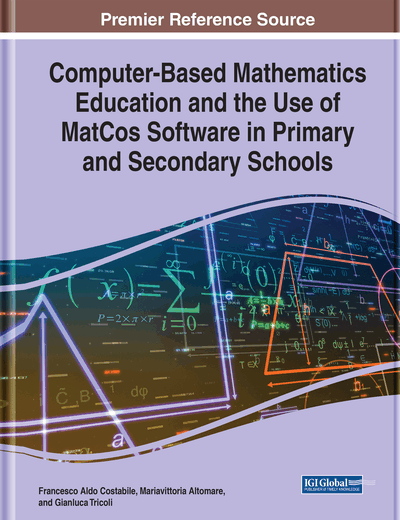# A Statistical-Probabilistic Path

DOI: 10.4018/978-1-7998-5718-1.ch009
OnDemand:
(Individual Chapters)
Available
\$29.50
No Current Special Offers

## Abstract

This chapter is borderline compared to the previous ones, since the topics are not really mathematical. In fact, the objectives and learning content of statistics and probability are different from those of mathematics. It also follows a methodological diversity of teaching. All this will be clarified below. However, the main focus of the goal, that is the possible contribution that the computer with the correct programming can give to the learning of the discipline, is no less evident and will be clearly delineated. In particular, graphical representations of statistical data will be widely examined. A TLS will also be schematized on the introduction of the concept of probability, in which the use of the computer with the MatCos 3.X environment will be very useful.
Chapter Preview

The time may not be very remote when it will be understood that for a complete initiation as an efficient citizen of one of the new great complex world-wide states that are now developing, it is as necessary to be able to compute, to think in averages and maxima and minima, as it is now to be able to read and write. ~H. G. Wells (1903, p. 204)

Top

## 1. Introduction

This chapter is slightly different compared to the rest of the book, as is highlighted in the title. In fact, our main intention is to show how it is concretely possible to support the teaching / learning of Mathematics in primary and secondary schools, with the computer and a programming environment which is appropriate to the context. The subject of Statistics is different from that of Mathematics in terms of objectives and methods. Statistics is a quantitative science, but the “statistical” way of thinking is different from the “mathematical” way, for at least two reasons:

• statistics cannot be separated from the context (contextualized data or numbers);

• the logic of statistical inference is not based on deduction, as in mathematics, but on induction: from the particular (what has been observed) to the general. This concept of induction is also very different from mathematical induction.

For Statistics, Mathematics has an instrumental role, which is that it provides the tools that allow for statistical analysis. These differences have implications for teaching methods:

• -

to teach Statistics well it is not enough to know Mathematics and statistical theory;

• -

it is essential to have real examples and know how to use them to involve students and lead them to develop critical judgment skills, to make decisions and forecasts in conditions of uncertainty;

• -

today we could add: it is also necessary to have adequate calculation tools for data processing and printing.

## Key Terms in this Chapter

Frequentist Approach: It claims that the probability of a random event denotes the relative frequency of occurrence of an experiment's outcome, when repeating the experiment.

MatCos 3.X: Programming environment oriented towards mathematics, aimed at high school.

Descriptive Statistics: Are brief descriptive coefficients that summarize a given data set, which can be either a representation of the entire or a sample of a population. Descriptive statistics are broken down into measures of central tendency and measures of variability (spread).

Diagram Statistic: A diagram which exhibits a simple analysis of statistical data in terms of percentages. The actual form of the diagram can vary; examples are the bar chart, the pie chart, the Circular diagram, the Histogram.

## Complete Chapter List

Search this Book:
Reset